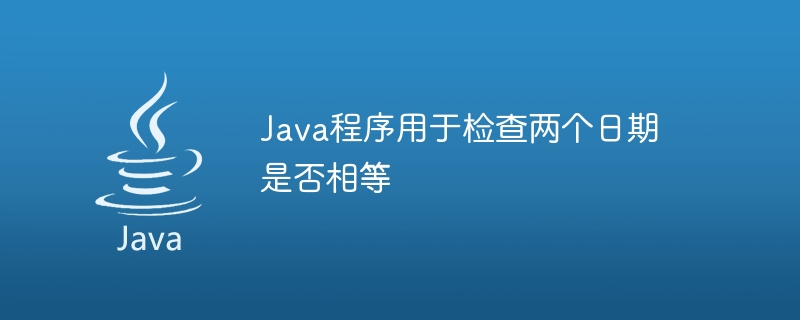# Java程序用于检查两个日期是否相等## 检查两个日期是否相等的Java程序

### compareTo()

Comparable 接口仅定义了一个名为“CompareTo”的方法，该方法提供了将类的对象与其自身进行比较的功能。当第一个日期对象等于传递的对象时，它返回 0，如果第一个日期对象更大，则返回正值，否则返回负值。

### 语法

```dateOne.compareTo(dateTwo);
```

### 语法

```dateOne.equals(dateTwo);
```

### 示例 1

```import java.time.*;
import java.util.*;
public class Main {
public static void main(String[] args) {
// initializing two unequal dates
LocalDate dateOne = LocalDate.parse("2021-01-20");
LocalDate dateTwo = LocalDate.parse("2023-06-01");
// checking both dates are equal or not
if(dateOne.equals(dateTwo)) {
System.out.println("Both dates are equal!");
} else {
System.out.println("Both dates are unequal!");
}
}
}
```

### 输出

```Both dates are unequal!
```

### 示例 2

```import java.text.SimpleDateFormat;
import java.util.Date;
public class Main {
public static void main(String[] args) throws Exception {
// creating instance of SimpleDateFormat
SimpleDateFormat timeformat = new SimpleDateFormat("yy/MM/dd");
// initializing two dates
Date dateOne = timeformat.parse("23/06/01");
Date dateTwo = timeformat.parse("23/06/01");
// checking both dates are equal or not
if(dateOne.compareTo(dateTwo) == 0) {
System.out.println("Both dates are equal");
} else {
System.out.println("Both dates are unequal!");
}
}
}
```

### 输出

```Both dates are equal
```

### 示例 3

```import java.text.SimpleDateFormat;
import java.util.Date;
public class Main {
public static void main(String[] args) throws Exception {
// creating instance of SimpleDateFormat
SimpleDateFormat timeformat = new SimpleDateFormat("yy/MM/dd");
// initializing two dates
Date dateOne = timeformat.parse("23/06/01");
Date dateTwo = timeformat.parse("23/06/01");
// checking both dates are equal or not
if(dateOne.equals(dateTwo)) {
System.out.println("Both dates are equal");
} else {
System.out.println("Both dates are unequal!");
}
}
}
```

### 输出

```Both dates are equal
```